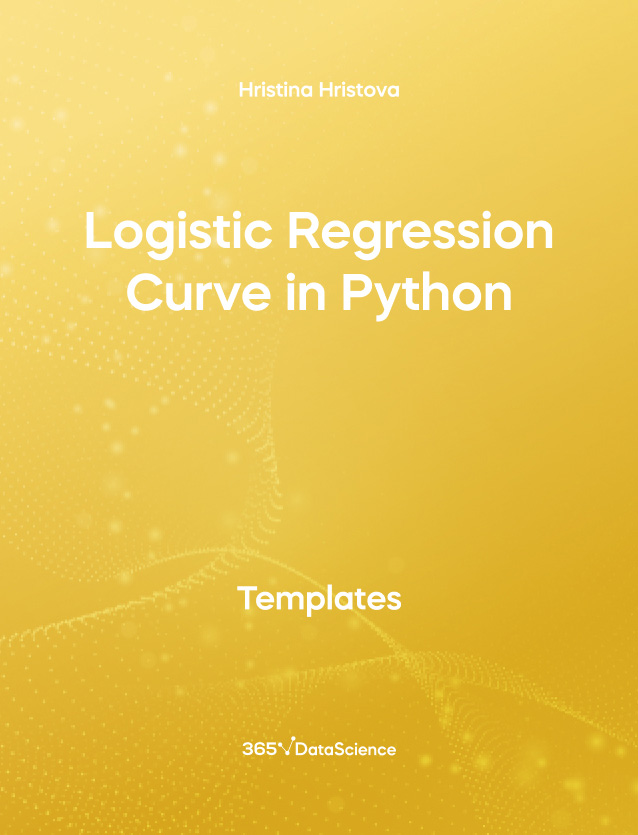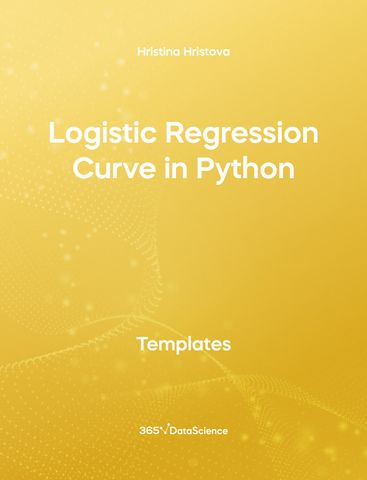# Logistic Regression Curve in PythonHristina Hristova

Course Author
In this template, we demonstrate how to create a logistic regression using statsmodels. Then, we plot the resulting regression curve onto the data points to visualize the result. Some other related topics you might be interested in are Logistic Regression with statsmodels in Python, Confusion Matrix with Statsmodels in Python, Model Accuracy in Python. You can now download the Python template for free. The Logistic Regression Curve in Python template is among the topics covered in detail in the 365 Data Science program.

## Who is it for

This is an open-access Python template that is going useful to Data Analysts, Data Scientists, Data Engineers, Web Developers, and anyone who is interested in learning how to plot a logistic regression curve.